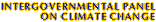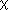Climate Change 2001: Working Group I: The Scientific BasisGet Javascript Other reports in this collection

### 5.4.2 Overall Uncertainty in Direct Forcing Estimates

To illustrate how the uncertainty associated with each of the factors determining aerosol direct forcing contribute to the overall uncertainty in forcing, we adopt a simple box-model of the overall change in planetary albedo. This approach follows that presented in Penner et al. (1994a), but is updated in several respects. For this model, the change in global average planetary albedo associated with anthropogenic aerosols is described as (Chylek and Wong, 1995):where,

Ta = atmospheric transmissivity above the main aerosol layer
Ac = global cloud fraction
Rs = global average surface albedo= upscatter fraction for isotropic incoming radiation
fb = hygroscopic growth factor for upscatter fraction
M = global mean column burden for aerosol constituent, (gm-2)
as = aerosol mass scattering efficiency, (m2g-1)
f(RH) = hygroscopic growth factor for total particle scattering
wo = single scattering albedo at ambient RH (assumed to be 80% for this analysis).

The key quantities that enter into the calculation of uncertainties are listed in Table 5.10, together with estimates of their 2/3 uncertainty range. Given these central values and uncertainties, the variance (and thus uncertainty) associated with the planetary albedo change (Dap) is determined by standard Taylor expansion of the function around the central values for the change in planetary albedo. Thus, with variances given by Si2:where the function cov(xi,xj) is the covariance of the variables in the argument and a variable subscript (i.e., i or j) implicitly requires summation from 1 to n where n is the number of variables. Significant covariances are found between b and as, b and fb, as and f(RH), and w0 and f(RH). For these variable pairs, Bravais-Pearson (linear) correlation coefficients were found to be -0.9, -0.9, 0.9 and 0.9, respectively. These were determined by sampling the probability distribution associated with each pair of variables to generate a large set of corresponding pairs of values. Linear regression analysis was then performed on these corresponding pairs to determine the linear correlation coefficient between the paired variables. The burden estimates in Table 5.10 are based on model calculations from the IPCC workshop. The uncertainty range was taken from the range in burdens from the models (assumed here to be a 2/3 uncertainty range) which may be a reasonable estimate for some of the structural uncertainty associated with different parametrization choices in the models (Pan et al., 1997). It includes both chemical quantities such as the fraction of emitted SO2 that is converted to sulphate aerosol and the mean residence time of the aerosol. The central emissions estimates are those specified in the model workshop (except where noted) and the uncertainties are those estimated in Section 5.2. The uncertainty range for the burden, M, used in evaluating the change in planetary albedo was calculated from the uncertainty in emissions together with the uncertainty in burden utilising the geometric concatenation procedure of Penner et al. (1994a).

 Table 5.10a: Factors contributing to uncertainties in the estimates of the direct forcing by aerosols associated with fossil fuels and other industrial processes and their estimated range. Note that optical parameters are for a wavelength of 550nm and are for dry aerosol.Quantity Central Value 2/3 Uncertainty RangeTotal emission of anthropogenic OC from fossil fuel burning (Tg/yr)a 20 10 to 30 Atmospheric burden of OC from fossil fuels (Tg)b 0.48 0.33 to 0.70 Total emission of anthropogenic BC from fossil fuel burning (Tg/yr) 7 4.67 to 10.5 Atmospheric burden of BC from fossil fuel burning (Tg)b 0.133 0.11 to 0.16 Total emission of anthropogenic sulfate from fossil fuel burning (Tg/yr) 69 57.5 to 82.8 Atmospheric burden of sulphate from fossil fuel burning (Tg S) 0.525 0.35 to 0.79 Fraction of light scattered into upward hemisphere,c 0.23 0.17 to 0.29 Aerosol mass scattering efficiency (m2g-1), asc 3.5 2.3 to 4.7 Aerosol single scattering albedo (dry), woc 0.92 0.85 to 0.97 Ta, atmospheric transmittance above aerosol layer d 0.87 0.72 to 1.00 Fractional increase in aerosol scattering efficiency due to hygroscopic growth at RH=80% 2.0 1.7 to 2.3 Fraction of Earth not covered by cloud d 0.39 0.35 to 0.43 Mean surface albedod 0.15 0.08 to 0.22Result: If central value is 0.6 Wm2 the 2/3 uncertainty range is from -0.1 to -1.0 Wm2a The central value estimated here was taken from Table 5.3, while the value used in intercomparison was 29. b The burden was estimated from the model intercomparison for total anthropogenic carbon taking the fraction associated with fossil fuels from the fraction of emissions associated with fossil fuels. c The central estimate and uncertainty range were calculated from the size distribution for polluted continental aerosols in Table 5.1. d Central estimate and uncertainty range adapted from values used by Penner et al. (1994a).

 Table 5.10b: Factors contributing to uncertainties in the estimates of the direct forcing by biomass burning aerosols and their estimated range. Note that optical parameters are for a wavelength of 550 nm and are for dry aerosol.Quantity Central Value 2/3 Uncertainty RangeTotal emission of anthropogenic OC from biomass burning (Tg/yr) 62.5 45 to 80 Atmospheric burden of OC from smoke (Tg)a 1.04 0.75 to 1.51 Total emission of anthropogenic BC from biomass burning (Tg/yr) 7 5.0 to 9.8 Atmospheric burden of BC from biomass burning (Tg)a 0.133 0.11 to 0.16 Fraction of light scattered into upward hemisphere,b 0.23 0.17 to 0.29 Aerosol mass scattering efficiency(m2g-1), asb 3.6 2.5 to 4.7 Aerosol single scattering albedo (dry), wob 0.89 0.83 to 0.95 Ta, atmospheric transmittance above aerosol layer c 0.87 0.72 to 1.00 Fractional increase in aerosol scattering efficiency due to hygroscopic growth at RH=80% 1.2 1.0 to 1.4 Fraction of Earth not covered by cloud c 0.39 0.35 to 0.43 Mean surface albedoc 0.15 0.08 to 0.22Result: If central value is 0.3 Wm2 the range is from 0.1 to 0.5 Wm 2a The burden was estimated from the model intercomparison for total anthropogenic carbon taking the fraction associated with biomass aerosols from the fraction of emissions associated with biomass aerosols. b The central estimate and uncertainty range were calculated from the size distribution for biomass regional haze in Table 5.1. c Central estimate and uncertainty range adapted from values used by Penner et al. (1994a).

Continues on next page